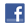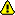New account
4 million accounts created!

• Home
• Report a bug

•

Learn German > German lessons and exercises > German test #8697> Other German exercises on the same topic: Numbers [Change theme] > Similar tests: - Numbers: 0-100 - Counting from 1 to 10 - Numbers from 20 to 100 - Numbers from 11 to 20 - Maths - Numbers - Counting from 100 to 1 000 - 100-100,000,000,000 > Double-click on words you don't understand

# Calculation : from 0 to 100

Give the result in letters.Twitter Share
German exercise "Calculation : from 0 to 100" created by micka with The test builder. [More lessons & exercises from micka]
Click here to see the current stats of this German testPlease log in to save your progress.

To insert special letters:

97 - 43 =

114 - 18 =

24 + 12 =

46 x 2 =

17 x 2 =

56 / 7 =

11 x 8 =

13 x 4 =

88 / 8 =

47 - 12 =

End of the free exercise to learn German: Calculation : from 0 to 100
A free German exercise to learn German.
Other German exercises on the same topic : Numbers | All our lessons and exercises